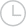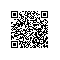Python创建一个街道地址表一码平川MACHEL2019-01-23 16:31:03470

M y s t r e
e t | r o a
d | d a o r
| t e e r t
s y M ? ? ?

givenNumber = input("What's your favourite number from 1 to 10?")
for row in range(0,int(len(strAddress)/givenNumber)):

for element in range(0,givenNumber):
print (splitAddress[element], end=' ')
print()

M y s t
r e e t
r o a
d

M y s t
M y s t
M y s t

Python取消 提交回答

• 一码平川MACHEL
2019-07-17 23:26:43

givenNumber = int(input("What's your favourite number from 1 to 10?"))

for row in range(lines_to_print):

for element in range(givenNumber):
if row*givenNumber + element < len(strAddress):
print(strAddress[row*givenNumber + element], end=' ')
else:
print('? ', end='')
print()

M y s t r e
e t | r o a
d | d a o r
| t e e r t
s y M ? ? ?

0 0